Next: MINIMUM MAXIMAL MATCHING Up: Covering and Partitioning Previous: MINIMUM FEEDBACK VERTEX SET   Index

### MINIMUM FEEDBACK ARC SET

• INSTANCE: Directed graph.
• SOLUTION: A feedback arc set, i.e., a subset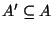such that A' contains at least one arc from every directed cycle in G.
• MEASURE: Cardinality of the feedback arc set, i.e.,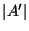.

• Good News: Approximable within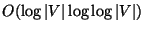.
• Comment: Transformation from MINIMUM FEEDBACK VERTEX SET . The generalized variation in which the input is extended with a subset S of vertices and arcs, and the problem is to find an arc set that contains at least one arc from every directed cycle that intersects S, is approximable within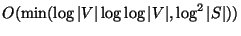. All these problems are approximable within 9/4 for planar graphs . The constrained variation in which the input is extended with a positive integer k and a subset S of A, and the problem is to find the feedback arc set of size k that contains the largest number of arcs from S, is not approximable within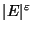for some. The complementary problem of finding the maximum set of arcs A' such thatis acyclic is approximable withinwhere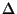is the maximum degree  and , it is APX-complete , and if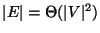it admits a PTAS .